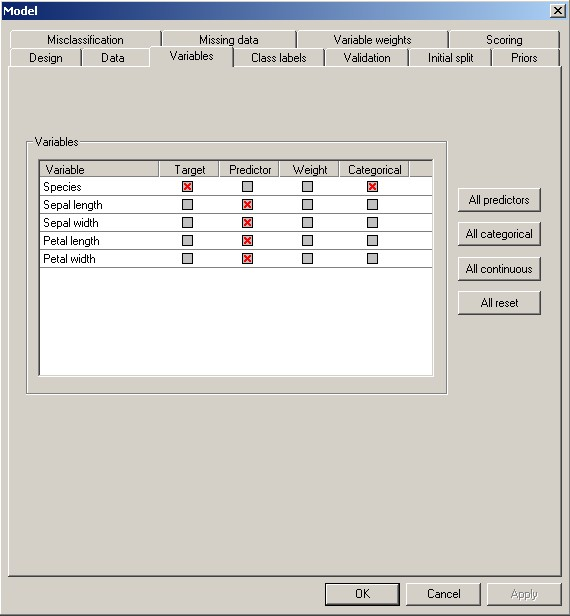# Classes and Types of Variables

Classes of Variables

You can specify three classes of variables when performing a decision tree analysis:

Target variable -- The “target variable” is the variable whose values are to be modeled and predicted by other variables. It is analogous to the dependent variable (i.e., the variable on the left of the equal sign) in linear regression. There must be one and only one target variable in a decision tree analysis.

Predictor variable -- A “predictor variable” is a variable whose values will be used to predict the value of the target variable. It is analogous to the independent variables (i.e., variables on the right side of the equal sign) in linear regression. There must be at least one predictor variable specified for decision tree analysis; there may be many predictor variables. If more than one predictor variable is specified, DTREG will determine how the predictor variables can be combined to best predict the values of the target variable.

Weight variable -- Optionally, you can specify a “weight variable”. If a weight variable is specified, it must a numeric (continuous) variable whose values are greater than or equal to 0 (zero). The value of the weight variable specifies the weight given to a row in the dataset. For example, a weight value of 2 would cause DTREG to give twice as much weight to a row as it would to rows with a weight of 1; the effect is the same as two occurrences of the row in the dataset. Weight values may be real (non-integer) values such as 2.5. A weight value of 0 (zero) causes the row to be ignored. If you do not specify a weight variable, all rows are given equal weight.

Types of Variables

Variables may have two types, continuous and categorical:

Continuous variables -- A continuous variable has numeric values such as 1, 2, 3.14, -5, etc. The relative magnitude of the values is significant (e.g., a value of 2 indicates twice the magnitude of 1). Examples of continuous variables are blood pressure, height, weight, income, age, and probability of illness. Some programs call continuous variables “ordered” or “monotonic” variables.

Categorical variables -- A categorical variable has values that function as labels rather than as numbers. Some programs call categorical variables “nominal” variables. For example, a categorical variable for gender might use the value 1 for male and 2 for female. The actual magnitude of the value is not significant; coding male as 7 and female as 3 would work just as well. As another example, marital status might be coded as 1 for single, 2 for married, 3 for divorced and 4 for widowed. DTREG allows you to use non-numeric (character string) values for categorical variables. So your dataset could have the strings “Male” and “Female” or “M” and “F” for a categorical gender variable. Because categorical values are stored and compared as string values, a categorical value of 001 is different than a value of 1. In contrast, values of 001 and 1 would be equal for continuous variables.

DTREG Variable Control Screen